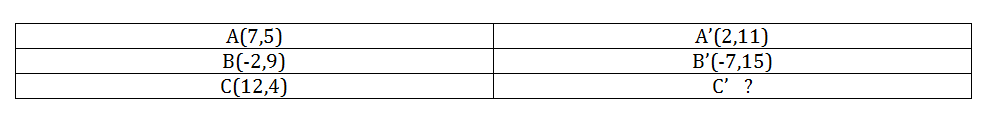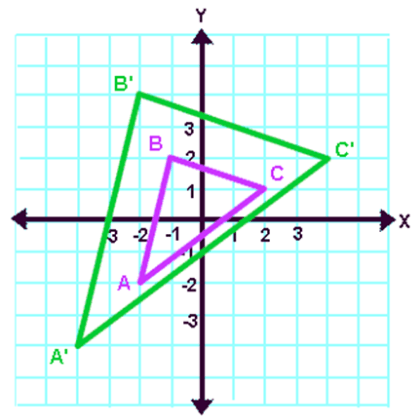Dilation
Reflection
Rotation
Random
Translation
100

Dilate the point (2,4) by a scale factor of 6

(12,24)

100

Reflect (-2,5) over the x-axis

(-2,-5)

100

Rotate (8,5) 90 degrees clockwise

(5,-8)

100

Triangle ABC has been translated to A'B'C'.  What is the coordinate for C'?(7,10)

100

Translate point (4,5) right 5 units and down 3 units

(9,2)

200

Dilate the point (7,21) by a scale factor of 1/7

(1,3)

200

Reflection (7,2) over y=x

(2,7)

200

Rotate (5,-1) 90 degrees counterclockwise

(1,5)

200

Find the scale factorK=2

200

(x,y) --> (x + 4 , y - 2)

A(2,-1)

Find A'

(6,-3)

300

The pre-image was (10,25) and the image was (6,15).  What is the scale factor?

k= 3/5

300

Reflect (5,1) over x = 3

(1,1)

300

Rotate (-6,-5) 270 degrees clockwise

(5,-6)

300

Point (8,5) is rotated 90 degrees clockwise and then reflected over y = -6.  Where is the point located?

(5,-4)

300

Write the rule for translating left 5 units and up 7 units

(x,y) --> (x-5 , y +7)

Click to zoom